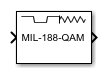# MIL-188 QAM Modulator Baseband

MIL-STD-188-110 B/C standard-specific quadrature amplitude modulation (QAM)

Since R2018b

•Libraries:
Communications Toolbox / Modulation / Digital Baseband Modulation / AM
Communications Toolbox / Modulation / Digital Baseband Modulation / Standard-Compliant

## Description

The MIL-188 QAM Modulator Baseband block modulates the input signal using MIL-STD-188-110 standard-specific quadrature amplitude modulation (QAM).

## Ports

### Input

expand all

Input signal, specified as a scalar, vector, or matrix. The input signal must be binary values or integers in the range [0, (M – 1)], where M is the Modulation order. This port is unnamed on the block.

Note

To process the input signal as binary elements, set the Input type parameter value to `Bit`. For binary inputs, the number of rows must be an integer multiple of log2(M). Groups of log2(M) bits in a column are mapped onto a symbol, with the first bit representing the MSB and the last bit representing the LSB.

Data Types: `single` | `double` | `int8` | `int16` | `int32` | `uint8` | `uint16` | `uint32` | `Boolean`

### Output

expand all

MIL-STD-188 standard-specific QAM modulated signal, returned as a complex scalar, vector, or matrix. The output signal dimensions depend on the specified Input type parameter value. This port is unnamed on the block.

Input typeDimensions of Output Signal
`Integer`The output signal has the same dimensions as the input signal.
`Bit`The number of rows in the output signal equals the number of rows in the input signal divided by log2(M), where M is the Modulation order.

## Parameters

expand all

Modulation order, M, specified as `16`, `32`, `64`, or `256`. The modulation order specifies the total number of points in the constellation of the output signal.

Constellation scaling preference, specified as:

• `As specified in standard` – The block scales the constellation based on specifications in the relevant standard .

• `Unit average power` – The block scales the constellation to an average power of 1 watt referenced to 1 ohm.

Input type, specified as `Integer` or `Bit`. To use `Integer`, the input signal must consist of integers in the range [0, (M – 1)]. To use `Bit`, the input signal must contain binary values, and the number of rows must be an integer multiple of log2(M), where M is the Modulation order.

Output data type, specified as `double` or `single`.

To plot the reference constellation, click the View Constellation button.

Type of simulation to run, specified as:

• `Code generation` –– Simulate the model using generated C code. The first time you run a simulation, Simulink® generates C code for the block. The C code is reused for subsequent simulations as long as the model does not change. This option requires additional startup time, but the speed of the subsequent simulations is comparable to `Interpreted execution`.

• `Interpreted execution` –– Simulate the model using the MATLAB® interpreter. This option shortens startup time and has a simulation speed comparable to ```Code generation```. In ```Interpreted execution``` mode, you can debug the source code of the block.

## Block Characteristics

 Data Types `Boolean` | `double` | `integer` | `single` Multidimensional Signals `yes` Variable-Size Signals `no`